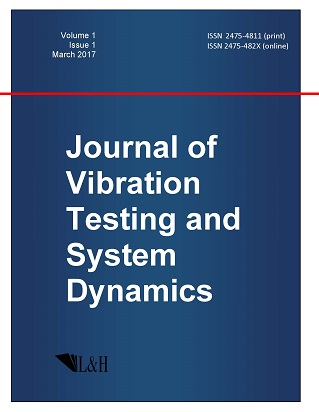ISSN: 2475-4811 (print)
ISSN: 2475-482X (online)
Journal of Vibration Testing and System Dynamics

C. Steve Suh (editor), Pawel Olejnik (editor),

Xianguo Tuo (editor)

Pawel Olejnik (editor)

Lodz University of Technology, Poland

Email: pawel.olejnik@p.lodz.pl

C. Steve Suh (editor)

Texas A&M University, USA

Email: ssuh@tamu.edu

Xiangguo Tuo (editor)

Sichuan University of Science and Engineering, China

Email: tuoxianguo@suse.edu.cn

Journal of Vibration Testing and System Dynamics 5(3) (2021) 279--283 | DOI:10.5890/JVTSD.2021.09.008

N.H. Ibragimov$^{1,2}$, M. Torrisi$^{3}$, R. Tracin 'a$^{3}$

$^{1}$ Center ALGA, Department of Mathematics and Natural Sciences, Blekinge Institute of Technology, Karlskrona, Sweden

$^{2}$ Laboratory Group Analysis of Mathematical models in Natural and Engineering Sciences", Ufa State Aviation Technical University, Ufa, Russia

$^{3}$ Dipartimento di Matematica e Informatica, Univerity of Catania, Italy

Abstract

In this paper we show some short notes concerned the nonlinear selfadjointess of a class of wave equations. These results, obtained together with prof. Ibragimov between the end of 2017 and January 2018, were the beginning of a wide study that remained incomplete.

References

1.   Ibragimov, N.H., Torrisi, M., and Tracin\a, R. (2010), Quasi self-adjoint nonlinear wave equations, J. Phys. A: Math. Theor., 43, 442001, (8pp).
2.   Ibragimov, N.H. (2006), Integrating factors, adjoint equations and Lagrangians, J. Math. Anal. Appl., 318, 742-757.
3.   Ibragimov, N.H. (2007), A new conservation theorem, J. Math. Anal. Appl., 333, 311-328.
4.   Ibragimov, N.H. (2011), Nonlinear self-adjointness and conservation laws, J. Phys. A: Math. Theor., 44, 432002.
5.   Sophocleous, C. and Tracin\{a}, R. (2008), Differential Invariants for quasi-linear and semi-linear wave-type equations, Appl. Math. Comput., 202 pp. 216-228.